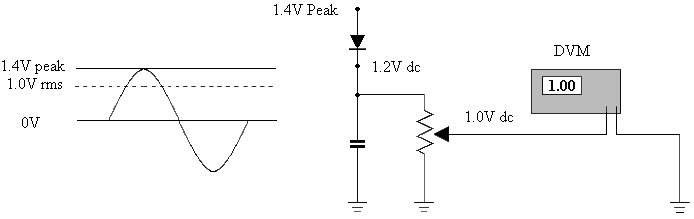# Appendix C - Voltage / Current Detector

C.1) Introduction

The Voltage / Current Detector is designed to be used in a coaxial transmission line and detects Peak Vector Sum Voltage and Peak Vector Difference Current. We make these specifically for the purpose of studying signal behaviour in coaxial cables but they could also be used as a simple RF power meter or for studying antennas.

A sine wave can be detected by a diode pump circuit as in Figure 1.1. If there is a signal input to the anode of the diode of 1.4 Volts peak (1 Volt rms) and the diode is a Schottky with a forward voltage drop of 0.2 Volts, then the DC level on the diode cathode will be 1.2 Volts. If a digital voltmeter is now connected to the output of the circuit and the potentiometer is adjusted to give a meter reading of 1 Volt. The dc output of 1.0 Volts now indicated by the meter, represents the rms voltage present at the anode of the diode.

Figure 1.1This circuit functions well, as long as there is an adequate input voltage to the diode. When the input voltage to the diode anode nears the voltage drop of the diode the output voltage drops disproportionately and the circuit becomes non-linear and closer to a square law. There are methods of forward biasing the diode in order to reduce these errors but it was considered more suitable to reduce the errors mathematically.

Measurements are made at known voltage levels and the results are used to plot a curve. A formula is then fitted to the curve in order to find the constants for the diode in use. During our measurements, we will use a signal generator as the RF source and a DMM to monitor dc voltages. Most modern RF signal generators are 50 Ohms and indicate their output level in dBm, although some can indicate in Volts. DMM's indicate in volts and diode data is normally presented in Volts. We will therefore, use Volts as the common units.

If it is necessary to convert the signal generator output from dBm to Volts, we start by finding the voltage at the reference level of 0 dBm:

0 dBm = 1 mW into 50 Ohms

From Ohms law  W = V2 / R

Change to Impedance  V2 = W x Z

Substitute reference level  Vref = (Wref x Z)^0.5  Formula 1.1

To find the voltage at a particular output power level:

From db = 20 x Log (V1/V2)

V1 = Alog(dB/20) x V2

Substitute required voltages:

Vout = ALog(dB/20) x Vref

Substituting Formula 1.1 for Vref :

Vout = ALog(dB/20) x (Wref x Z)^0.5  Formula 1.2

As an example, calculate the output voltage at +13 dBm:

V = ALog(13/20) x (0.001 x 50)^0.5

V = 4.467 x 0.2236 = 0.9988 Volts rms

We can now use Formula 1.2 (or the indicated signal generator output voltage) in a spreadsheet to give a range of signal generator output voltages related to one dB steps from - 10 dBm to +13 dBm. The generator output level in dB is placed in ascending order in the first column (A). The voltage calculation and its result is placed in the second column (B). This has been done for you in linearity-spreadsheet-01.xls, but it is better experience if you generate your own spreadsheets.

The Voltage and Current Detector is connected directly to the output of the Signal Generator, or with an adaptor and is terminated with a 50 Ohm load as shown in Figure 1.2. The Signal Generator output is set to +13 dBm (or 1 Volt rms) at a frequency of 50 MHz. The DMM is connected to the Voltage and Current Detector, voltage output and should indicate approximately 1 Volt.

Figure 1.21    2      Page Number

Transmission Line Index

Technical Article Index